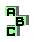VECTEN SQUARES

 d Vecten points r169 r170 altitudes r178 altitudes d Grebe triangle r339 point X(376),centroid r340 symmedian point, pedal triangle, homothetic triangles r469 circumcircle, Vecten points r470 Vecten points, circumcenter r492 Brocard line, radical center r493 circumcenter, symmedian point, homothetic triangles r494 antipedal triangle, centroid, point X(376), homothetic triangles r495 symmedian point, circumcenter, Euler line, homothetic triangles r505 centroid, symmedian point r506 Vecten points, anticomplement r538 antimedial triangle, Kiepert hyperbola r638 altitude feet r639 altitudes r736 Vecten points, circumcenter, complement r737 Vecten points, circumcenter r738 Kiepert hyperbola r797 incircle, ninecenter, radical center r1546 Vecten points r1695 incenter